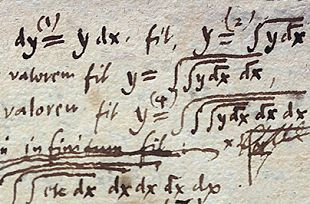Search IntMath
Close

450+ Math Lessons written by Math Professors and Teachers

5 Million+ Students Helped Each Year

1200+ Articles Written by Math Educators and Enthusiasts

Simplifying and Teaching Math for Over 23 Years

# 9. Integration Using Tables of Integrals

by M. Bourne

### Leibniz's original integral notationGottfried Leibniz's original notation for integrals included an overbar, similar to what we use with square roots today. [Image source]

A lot of the integrals in this section can easily be done using a Table of Integrals. (Of course, use a computer to do integrals whenever you can!)

Points to note:

• It's very important to understand the substitutions u and du (which is the case for most integrations)
• Make sure you use the correct formula!! Some are very similar to others.
• Even if you are supplied with a table of integrals in examinations, learn as many as you can, and especially learn the conditions that apply. Too many students try to find the right one in the table, but have no idea what they are doing!

### Example using Table of Integrals

Find the following integral, using table of integrals:

inte^(2x)\ sin 3x\ dx

We recognize this is the required formula:

inte^(au)\ sin bu\ du =(e^(au)(a\ sin bu-b\ cos bu))/(a^2+b^2)+K

For this example, we use:

a = 2

b = 3

u = x

So

inte^(2x)sin 3x\ dx=(e^(2x)(2\ sin 3x-3\ cos 3x))/(2^2+3^2)+K

=(e^(2x)(2\ sin 3x-3\ cos 3x))/13+K

There are some more examples of using the Table of Integrals in the section, Integration by Reduction Formulae.

## Problem SolverThis tool combines the power of mathematical computation engine that excels at solving mathematical formulas with the power of GPT large language models to parse and generate natural language. This creates math problem solver thats more accurate than ChatGPT, more flexible than a calculator, and faster answers than a human tutor. Learn More.